# Understanding the Text Formula Builder

The dynamic model builder uses a free text entry to apply filters and formulaic logic. The main reason for this is flexibility. With a text input we can apply mathematical operators to the Variables created.

### Compare Operators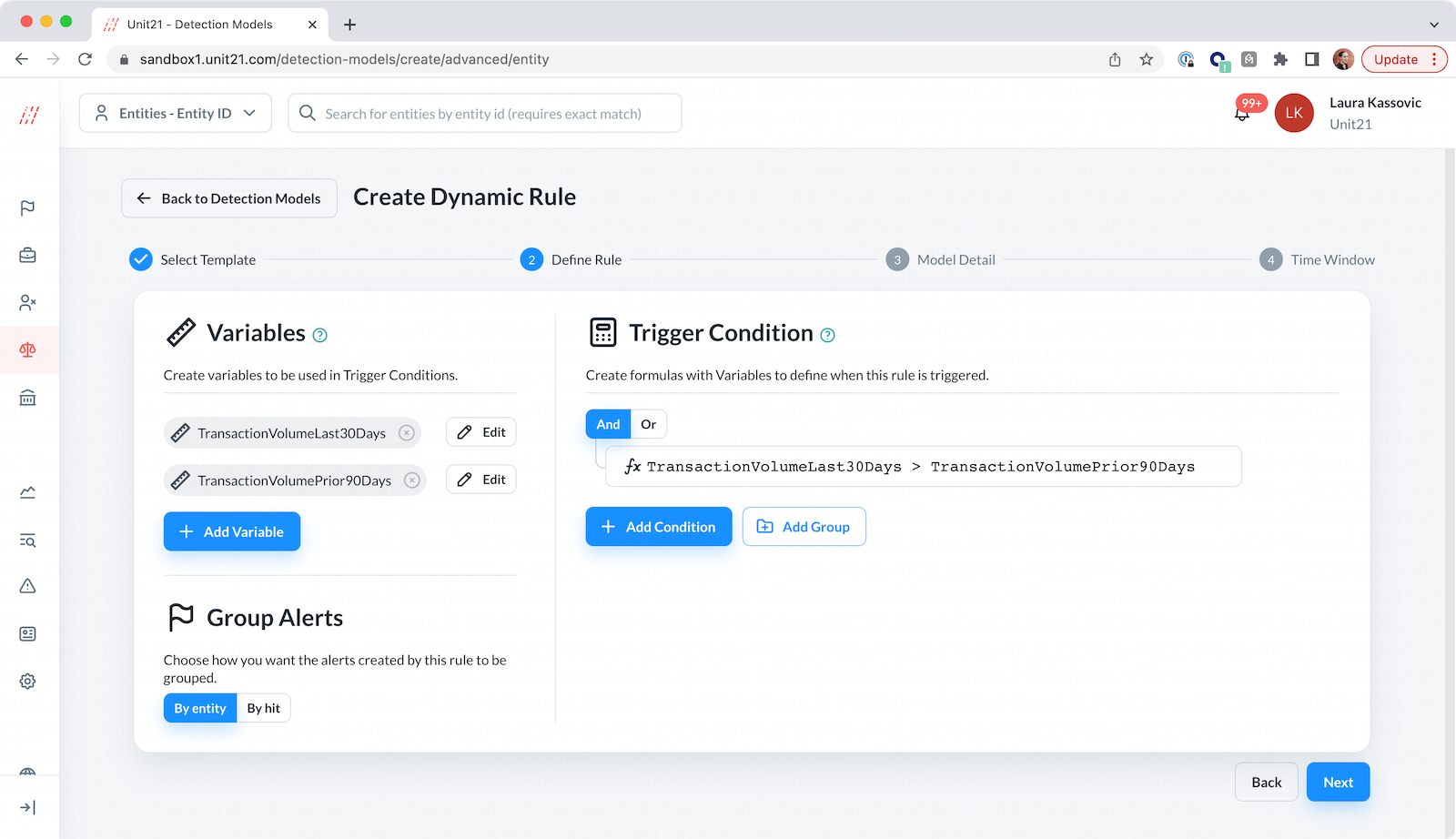Symbol

Name

`!=`

Not equal

`==`

Equal

`>=`

Greater than or equal to

`<=`

Less than or equal to

`<`

Less than

`>`

Greater than

`<=`

Less than or equal to

`<`

Less than

`>`

Greater than

`LIKE`

SQL-style LIKE
Operates on strings. Wildcard syntax is standard SQL % (e.g. entity.name LIKE 'FirstName %')

### Array Compare Operators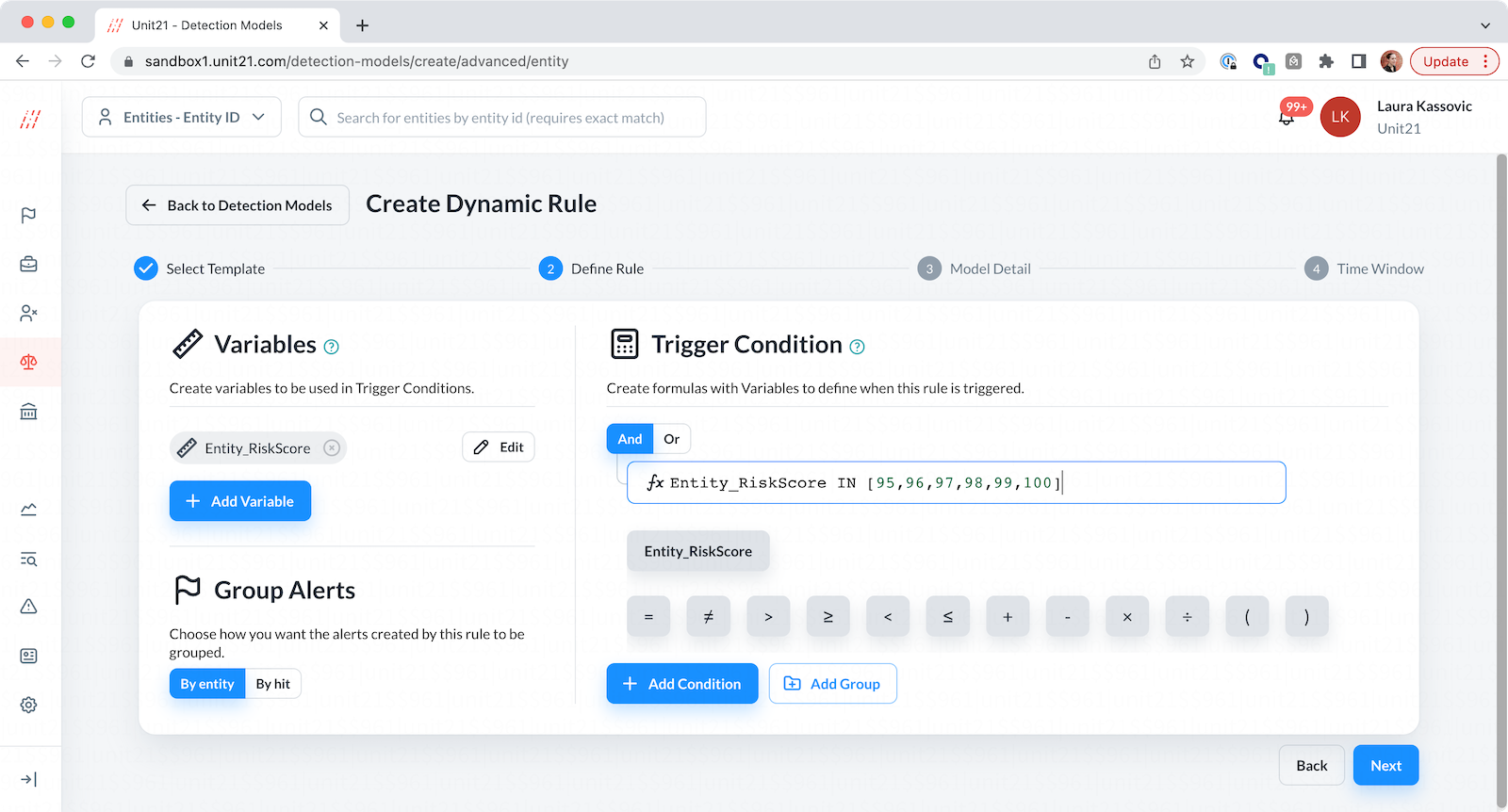Operator

Example

IN

IN [new, vip]

NOT_IN

NOT_IN [new, vip]

### Math Operators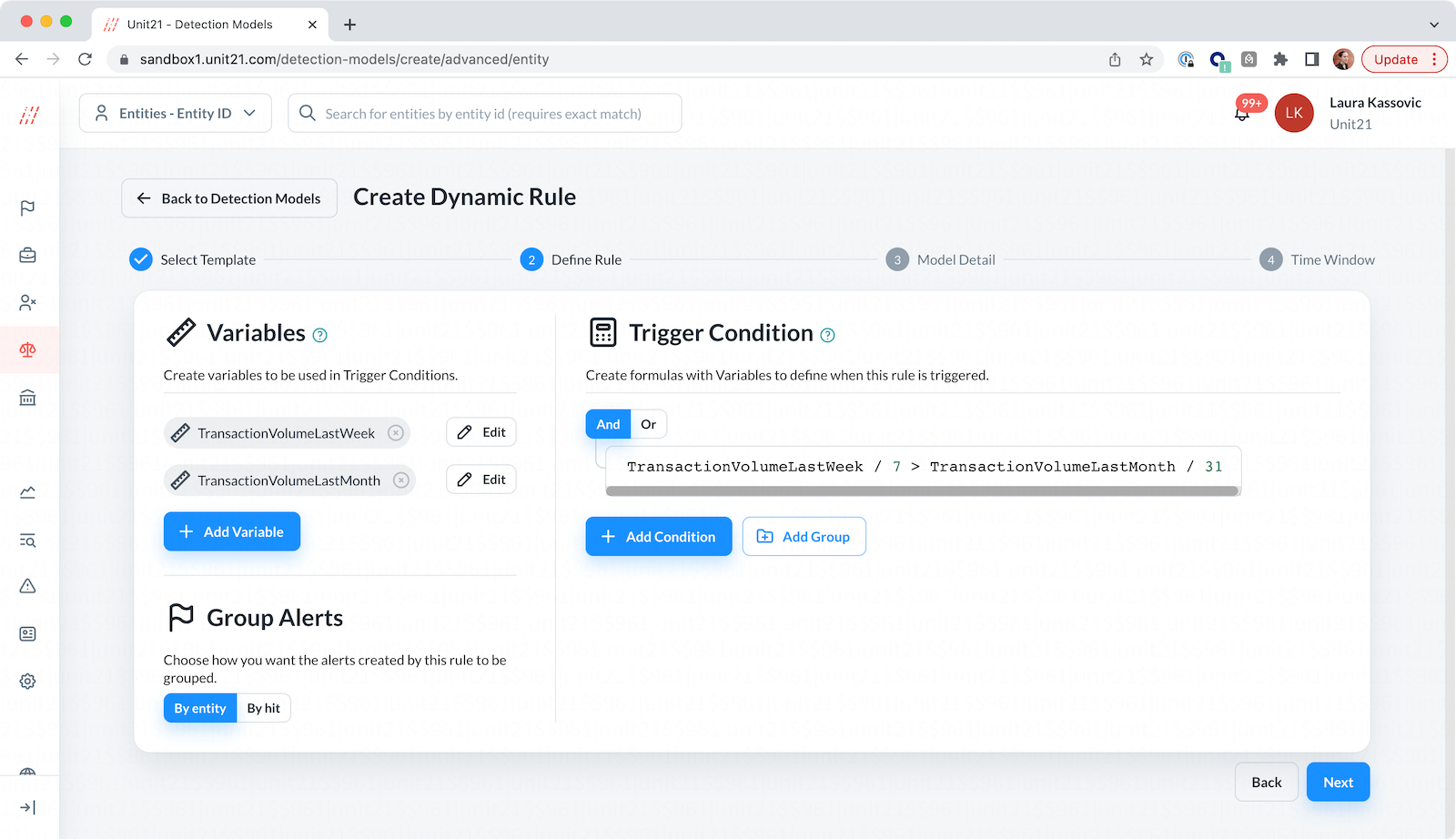Symbol

Name

`*`

Multiply

`+`

`-`

Subtract

`/`

Divide

### String Functions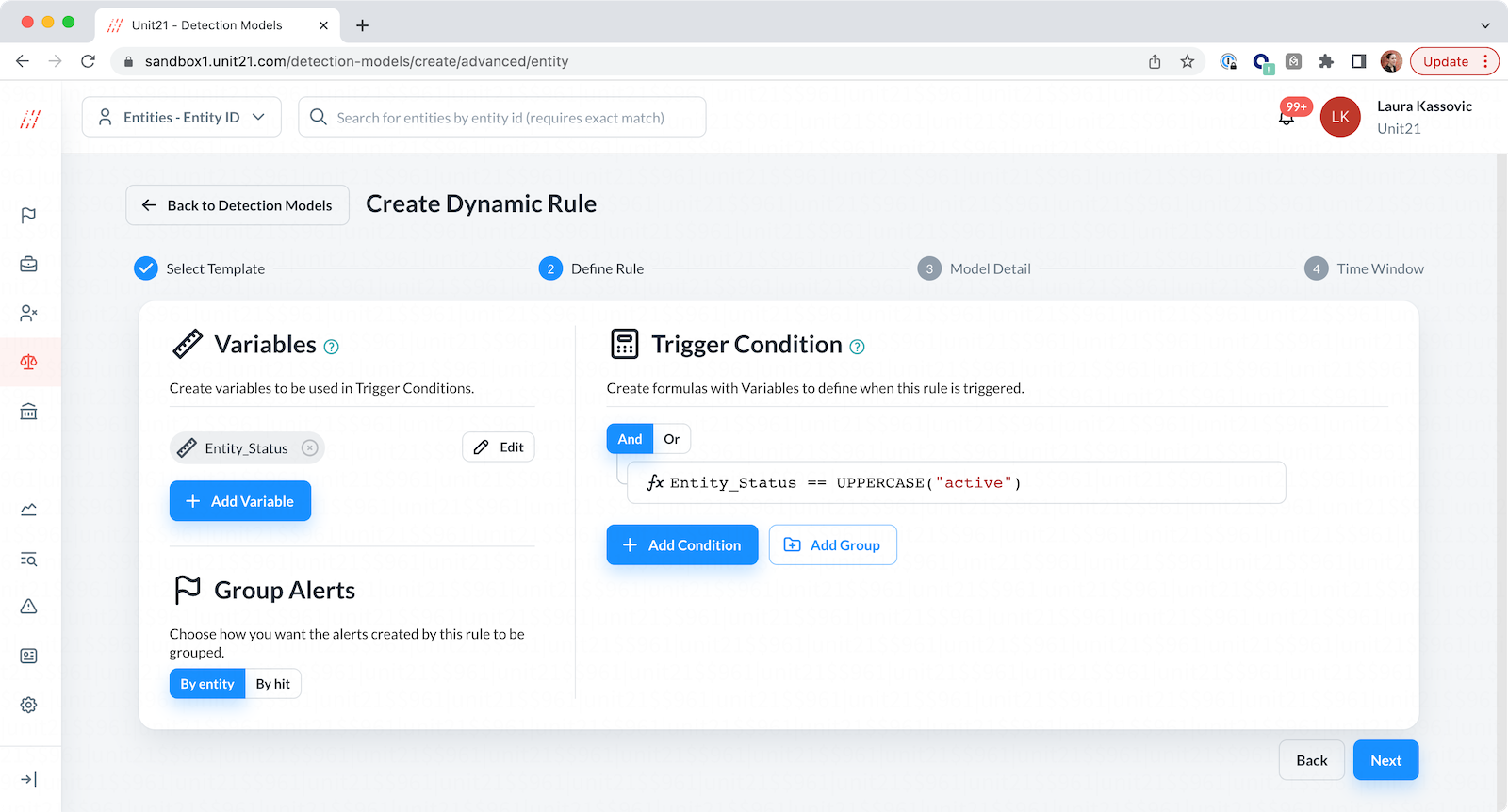Symbol

Description

UPPERCASE(string)

Convert string to uppercase

LOWERCASE(string)

Convert string to lowercase

CAPITALIZE(string)

Convert word-initial letters to caps

TRIM(string)

### Numeric Functions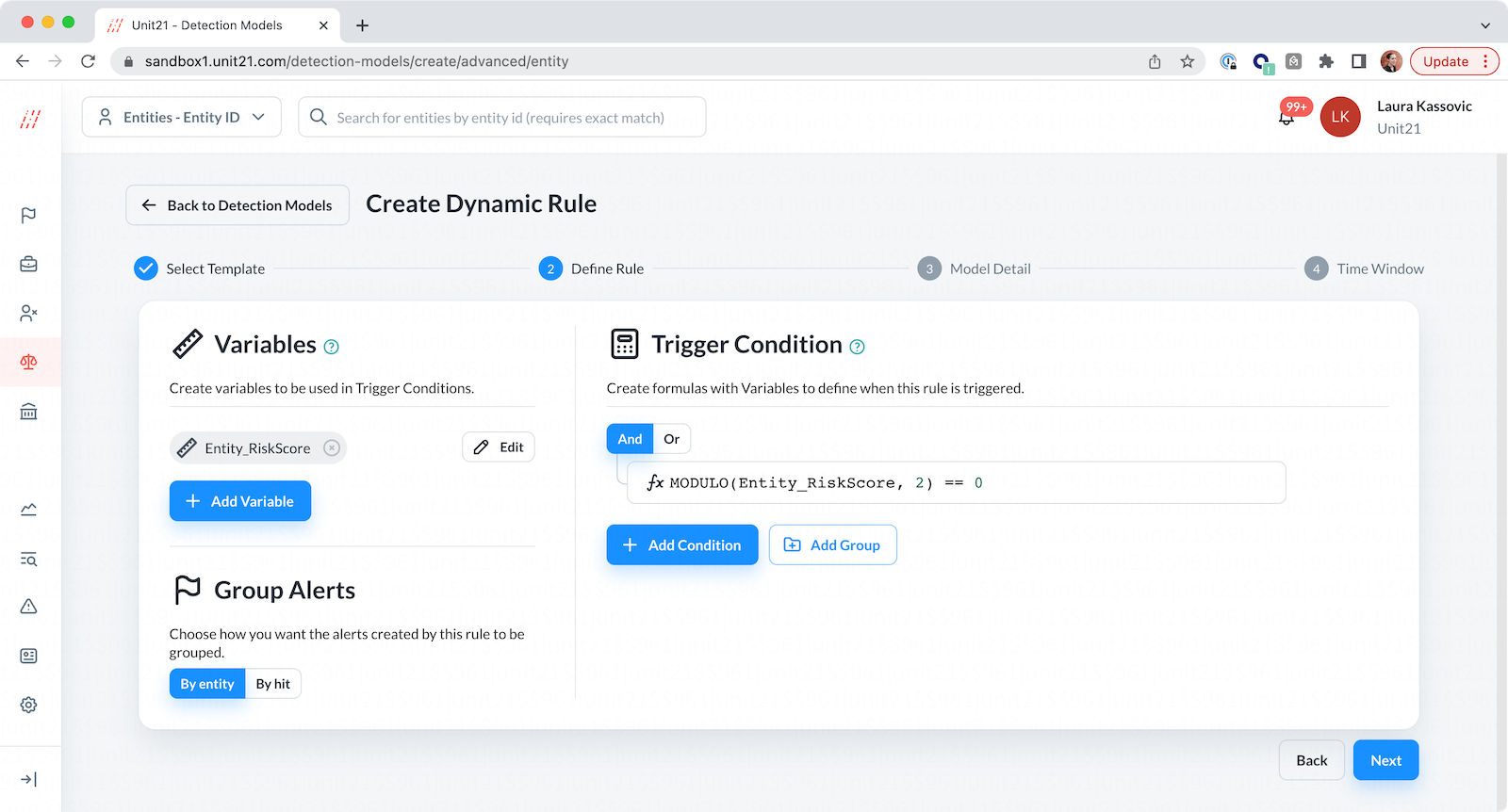Symbol

Description

ABS(x)

Absolute value of x

SQRT(x)

Square root of x

EXP(base, n)

raise to the power of

MODULO(a, b)

a mod b

LN(x)

Natural logarithm of x

LOG(x)

Base-10 log of x

### Time Compare Operators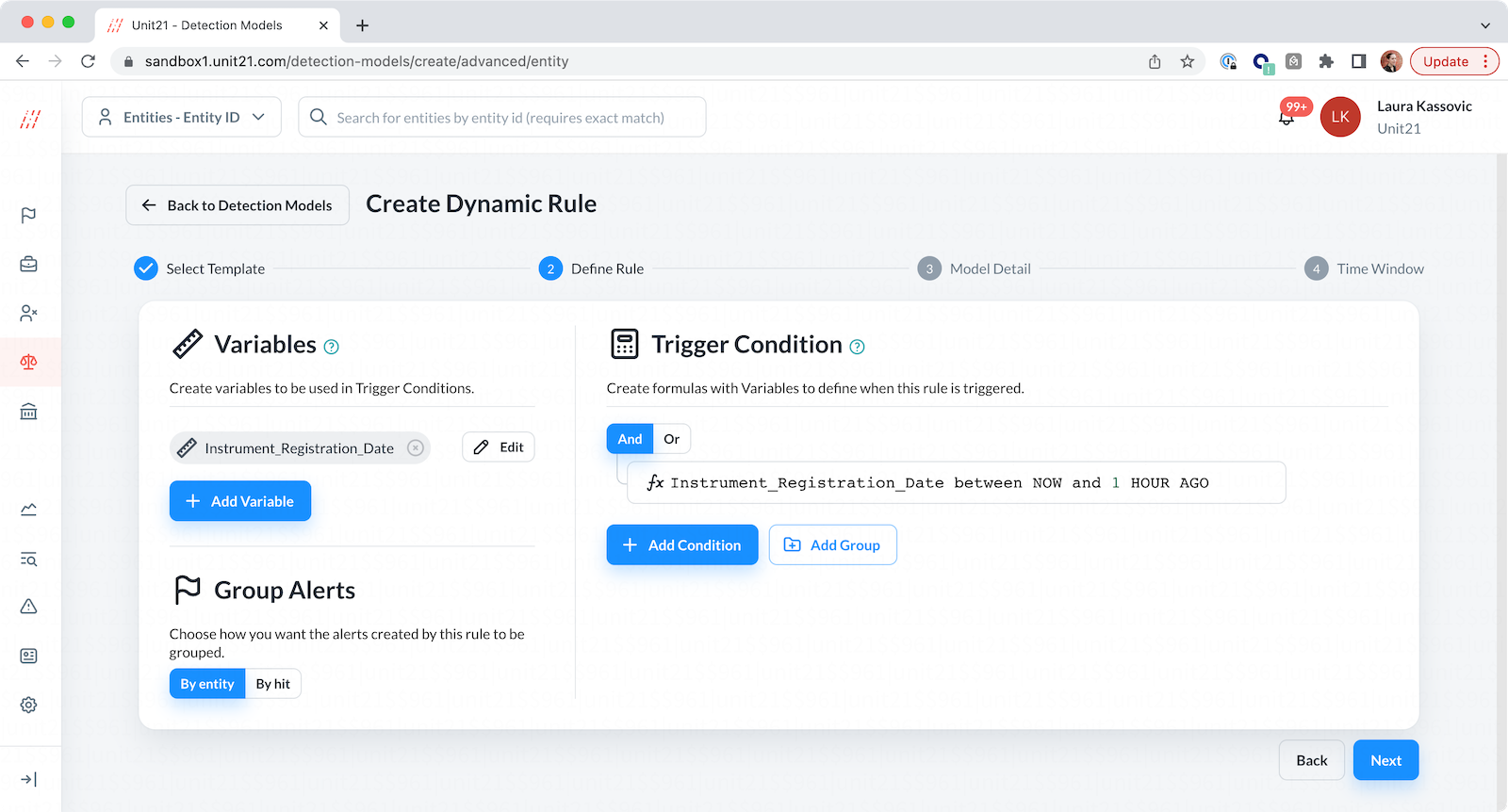Operator

Example

between

between 10 MIN AGO and 1 HOUR AGO

NOW

between NOW and 1 HOUR AGO

and

between NOW and 2 HOUR AGO

### Date Operators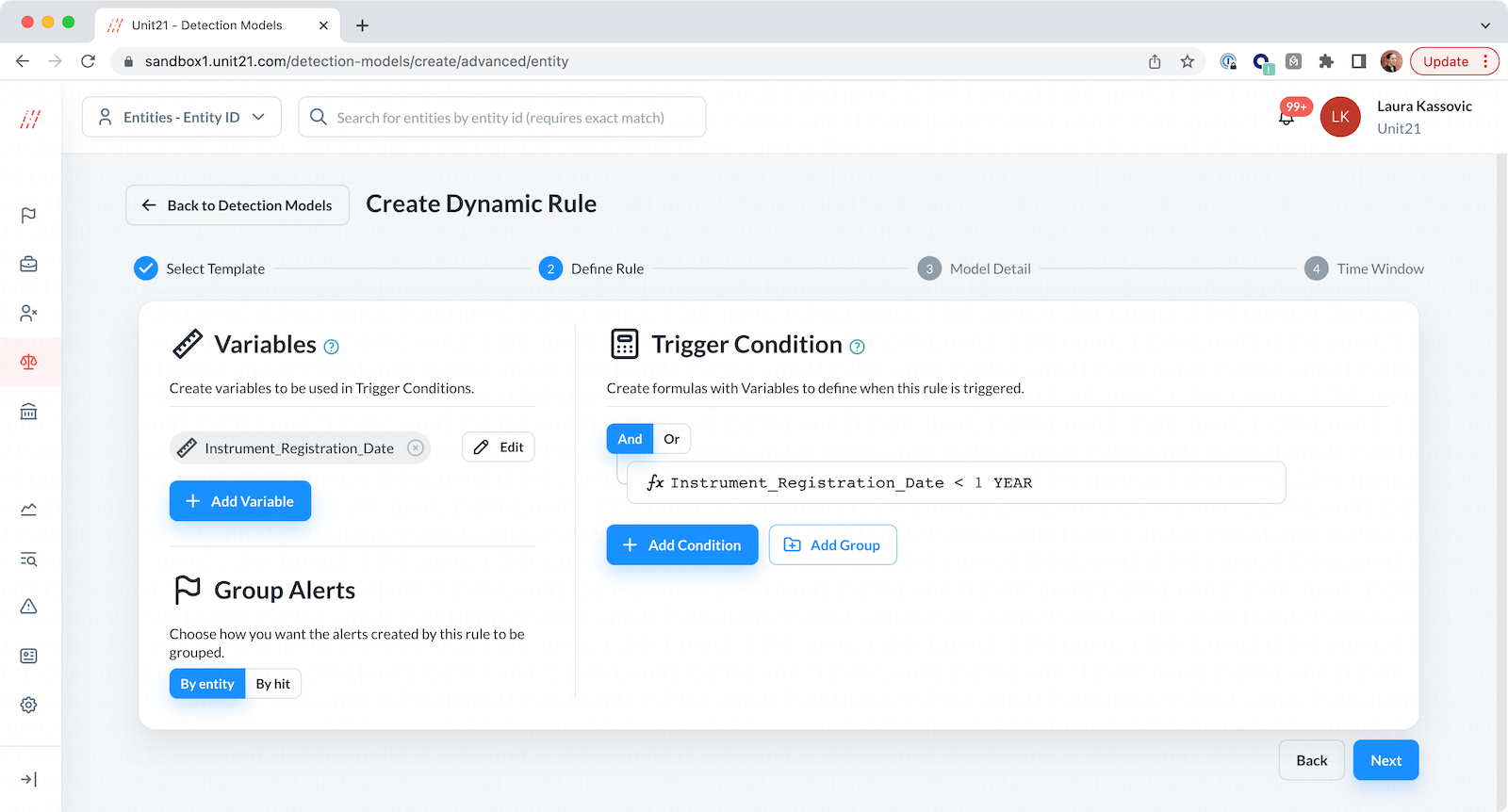Operator

Description

SECOND

seconds

MINUTE

minutes

DAY

days

HOUR

hours

WEEK

weeks

MONTH

months

YEAR

years

### Duration Operators:

Includes everything in Date Operators with the Keyword “AGO” appended at the end. e.g. YEAR AGO (edited).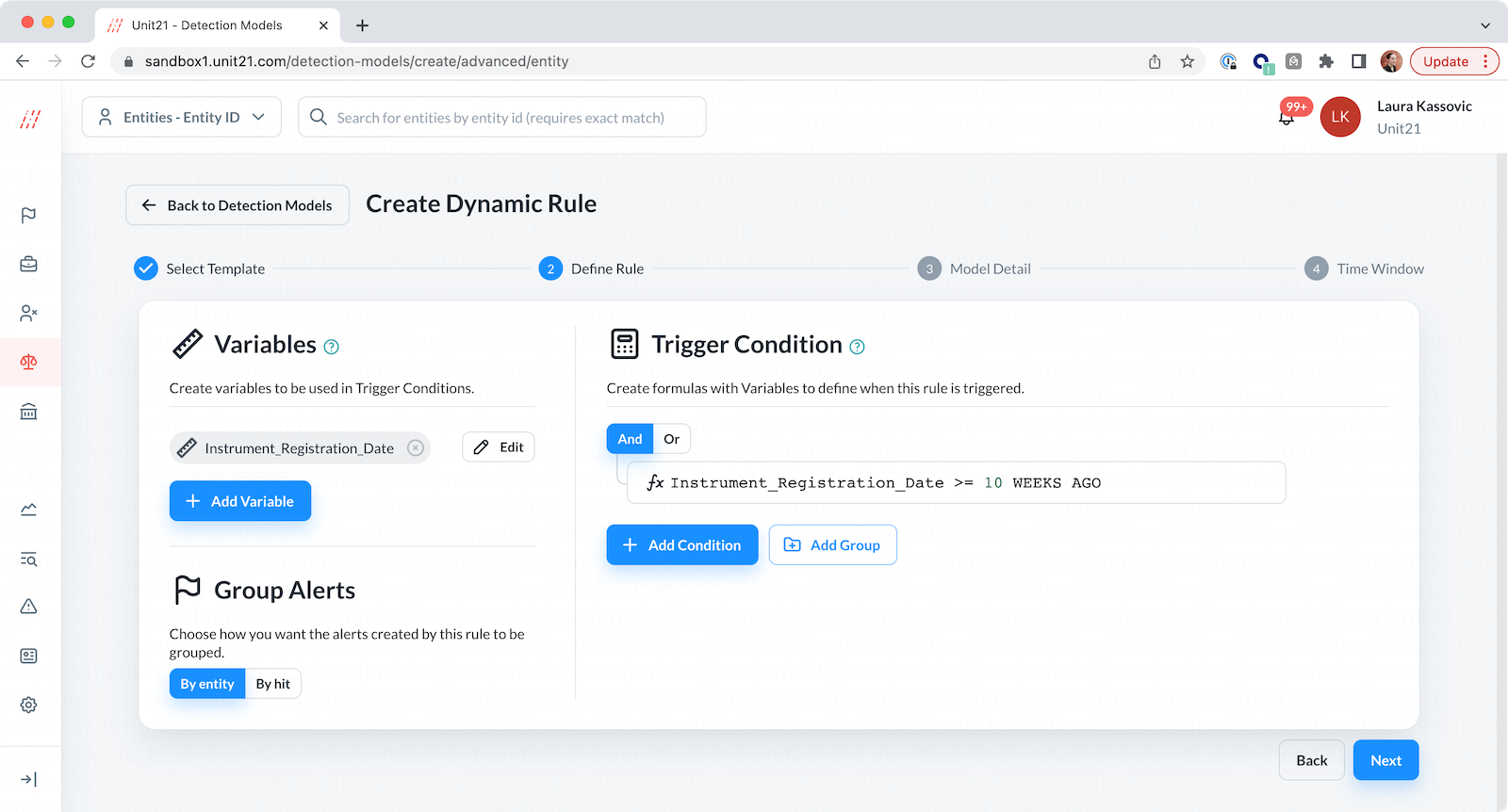Operator

Example

SECOND

10 SECOND AGO

MINUTE

10 MINUTE AGO

DAY

10 DAY AGO

HOUR

10 HOUR AGO

WEEK

10 WEEK AGO

MONTH

10 MONTH AGO

YEAR

10 YEAR AGO